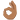# Pulley and two masses

## Homework Statement## The Attempt at a Solution

[/B]
When the right mass moves horizontally towards right , the verticle force acting on it is Mg - Tcosϑ , where ϑ is the angle which right string makes with verticle . The left mass experiences a force Mg - T . Since verticle force experienced by right mass is more , it moves closer to ground .Hence B) should be correct .

Is that right ?

#### Attachments

• 43.4 KB Views: 329

Related Introductory Physics Homework Help News on Phys.org
BvU
Homework Helper
2019 Award

•Chestermiller
Motion of masses in vertical direction are governed by respective net forces acting on them in vertical direction .The corresponding net forces have been mentioned in the post .

Is there anything more you would like me to explain ?

BvU
Homework Helper
2019 Award
Yes. I don'see the speed that is imparted to the mass appearing in your reasoning. So if the speed is zero, does it stilll apply ?
And you don't say what T is either ....

Did you try to do the experiment ?

And you don't say what T is either ....
Tension in the string connecting the two masses .
I don'see the speed that is imparted to the mass appearing in your reasoning. So if the speed is zero, does it stilll apply ?
Why would speed appear in force equations ?

We may consider any arbitrary horizontal speed being imparted to the right mass .

Chestermiller
Mentor
Tension in the string connecting the two masses .

Why would speed appear in force equations ?

We may consider any arbitrary horizontal speed being imparted to the right mass .
Please write down your force balance in the horizontal direction for the mass on the right.

Please write down your force balance in the horizontal direction for the mass on the right.
Tsinθ = Max

T is tension in the string and θ is angle which right string makes with vertical .

Chestermiller
Mentor
At the instant after the mass on the right has received its horizontal velocity, what is the angle theta, and what is its centripetal acceleration with respect to the point of contact of the string with the pulley?

•Jahnavi
what is the angle theta
zero .
what is its centripetal acceleration with respect to the point of contact of the string with the pulley?
v2/L

L is half length of string .

Chestermiller
Mentor
zero .

v2/L

L is half length of string .
OK. So, initially the vertical force balance on the right mass should really be: $$T-mg=m\frac{V_h^2}{L}+m\frac{dV_v}{dt}$$where $V_h$ is the initial horizontal velocity of the right mass and $\frac{dV_v}{dt}$ is the rate of change of its vertical velocity component.

•Jahnavi
Ok . What should be the next step in the analysis ?

Chestermiller
Mentor
Ok . What should be the next step in the analysis ?
Write the force balance on the left mass.

Write the force balance on the left mass.
T - Mg = May

Chestermiller
Mentor
T - Mg = May
So, what do you get if you subtract the two equations, recognizing that, for the left mass, $a_y$ is the same as $-dV_v/dt$ for the right mass?

•Jahnavi
So, what do you get if you subtract the two equations, recognizing that, for the left mass, $a_y$ is the same as $-dV_v/dt$ for the right mass?
$\frac{dV_v}{dt} = - \frac{V_h^2}{2L}$

Chestermiller
Mentor
$\frac{dV_v}{dt} = - \frac{V_h^2}{2L}$
Good. So what does this tell you about the initial vertical movement of the right- and left masses?

Last edited:
$\frac{dV_v}{dt} = - \frac{V_h^2}{2L}$
Lengthening and shortening of a string is given by the expression $\frac{dV_v}{dt}$ .Isn't it same for both the masses ?

Chestermiller
Mentor
Lengthening and shortening of a string is given by the expression $\frac{dV_v}{dt}$ .Isn't it same for both the masses ?
No. They are moving in opposite directions. The total length of string is constant, so the length of string on the right gets longer, and the length of string on the left gets shorter.

•Jahnavi
Bestfrog
Can I ask you where did you take this problem?

$\frac{dV_v}{dt} = - \frac{V_h^2}{2L}$
Good. So what does this tell you about the initial vertical movement of the right- and left masses?
Since you took positive upwards and from the above expression of vertical acceleration of right mass has a negative sign , it's initial acceleration is downwards .Likewise acceleration of left mass is positive means it's acceleration is upwards .

Right ?

Chestermiller
Mentor
Since you took positive upwards and from the above expression of vertical acceleration of right mass has a negative sign , it's initial acceleration is downwards .Likewise acceleration of left mass is positive means it's acceleration is upwards .

Right ?
right.

•Jahnavi
Can I ask you where did you take this problem?
Don't know the source .It was given in one of the Mechanics Practise sheets.

right.
Thank you so much. Your analysis was amazing.

Last edited:
So , basically the left mass moves upwards and the right mass moves downwards and rightwards simultaneously .

Right ?

Could you point out the exact flaw in my reasoning in first post ?

In light of your analysis , even though my initial attempt looks odd , I can't clearly see the exact problem with that reasoning .